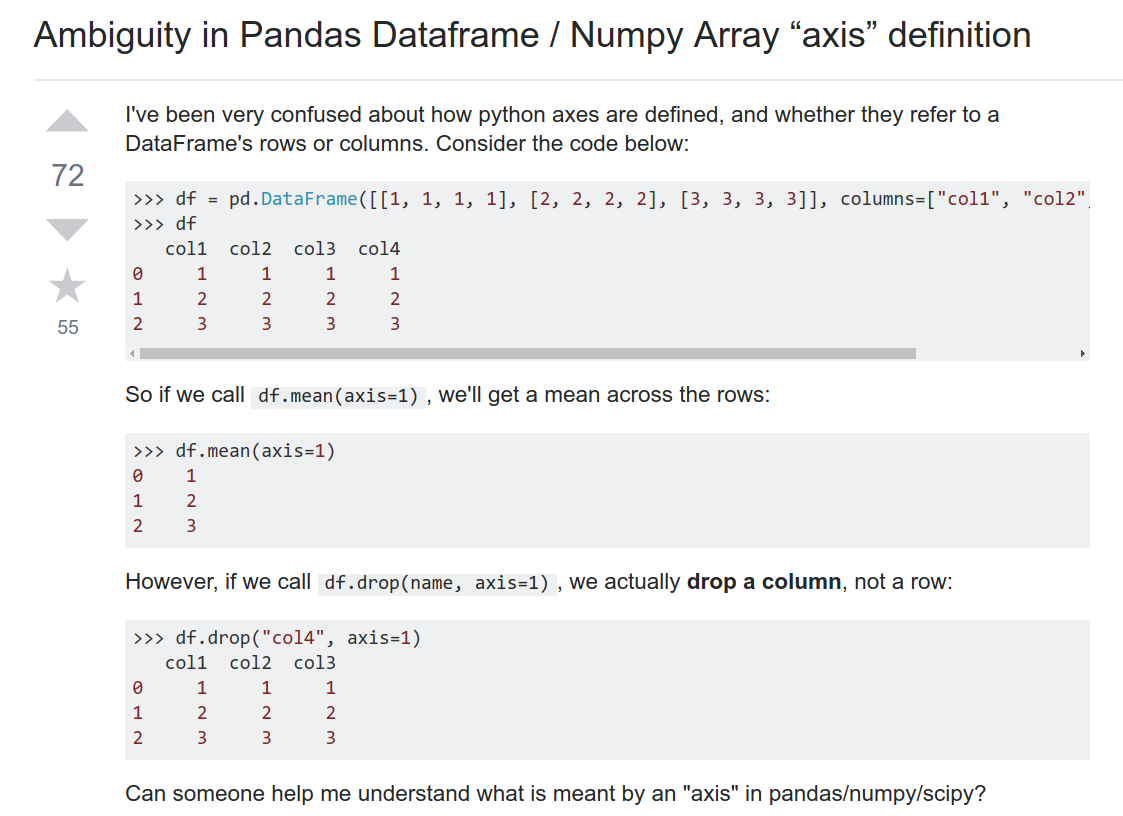1 2 3 4  In : import numpy as np In : a = np.arange(12).reshape(3,4) In : b = np.arange(12).reshape(4,3)

  1 2 3 4 5 6 7 8 9 10  In : print(a) [[ 0 1 2 3] [ 4 5 6 7] [ 8 9 10 11]] In : print(b) [[ 0 1 2] [ 3 4 5] [ 6 7 8] [ 9 10 11]]

  1 2 3 4 5 6 7 8 9 10 11 12 13  # axis = 0 是行 In : np.mean(a, axis=0) Out: array([4., 5., 6., 7.]) In : np.mean(b, axis=0) Out: array([4.5, 5.5, 6.5]) # axis = 1 是列 In : np.mean(a, axis=1) Out: array([1.5, 5.5, 9.5]) In : np.mean(b, axis=1) Out: array([ 1., 4., 7., 10.])It’s perhaps simplest to remember it as 0=down and 1=across.

This means:

• Use axis=0 to apply a method down each column, or to the row labels (the index).
• Use axis=1 to apply a method across each row, or to the column labels.

It’s also useful to remember that Pandas follows NumPy’s use of the word axis. The usage is explained in NumPy’s glossary of terms:

Axes are defined for arrays with more than one dimension. A 2-dimensional array has two corresponding axes: the first running vertically downwards across rows (axis 0), and the second running horizontally across columns (axis 1). [my emphasis]

So, concerning the method in the question, df.mean(axis=1), seems to be correctly defined. It takes the mean of entries horizontally across columns, that is, along each individual row. On the other hand, df.mean(axis=0) would be an operation acting vertically downwards across rows.

Similarly, df.drop(name, axis=1) refers to an action on column labels, because they intuitively go across the horizontal axis. Specifying axis=0 would make the method act on rows instead.

  1 2 3 4 5 6 7 8 9 10 11 12  In : print(a) [[ 0 1 2 3] [ 4 5 6 7] [ 8 9 10 11]] In : np.mean(a,axis=0) Out: array([4., 5., 6., 7.]) # 在 0 也就是行上上下操作，行全部压缩没了，上下求均数，所以剩下每一列一个均数 In : np.mean(a,axis=1) Out: array([1.5, 5.5, 9.5]) # 在 1 也就列上所有操作，所以列压缩没了，左右求均数，所以剩下每一行一个均数

  1 2 3 4 5 6 7 8 9 10 11 12 13 14 15 16  In : np.split(a, 2, axis=1) Out: [array([[0, 1], [4, 5], [8, 9]]), array([[ 2, 3], [ 6, 7], [10, 11]])] In : np.split(b, 2, axis=0) Out: [array([[0, 1, 2], [3, 4, 5]]), array([[ 6, 7, 8], [ 9, 10, 11]])] # 为了排版便于阅读我在结果部分加了换行，但是内容没有改动